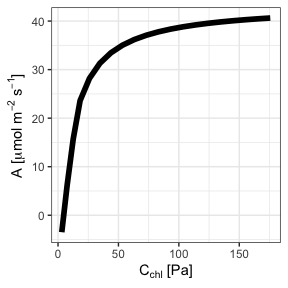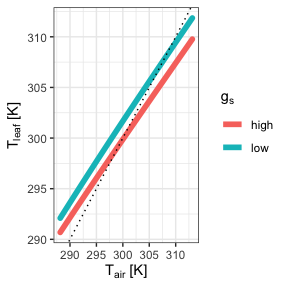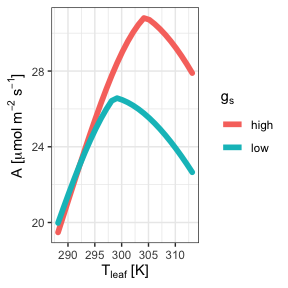Introduction to the photosynthesis package

2021-06-30

The photosynthesis package simulates photosynthetic rate given a set of leaf traits and environmental conditions by solving the Farquhar-von Caemmerer-Berry C3 biochemical model. There are two main steps to using photosynthesis:

1. define leaf parameters, environmental parameters, temperature response parameters, and physical constants; and
2. solve for the chloroplastic CO2 concentration that balances CO2 supply and demand (photo and photosynthesis for single and multiple parameter sets, respectively).

In this vignette, I’ll show you how to:

• run a minimum worked example using default parameters
• replace default parameters
• simulate photosynthetic rate along a gradient of CO$$_2$$ concentrations ($$A-C_\mathrm{c}$$ curve)

Minimum worked example

You can use the default parameter settings and simulate photosynthetic rate in a single leaf using the make_*() functions and photo().

library(dplyr)
library(magrittr)
library(photosynthesis)

# Leaving the make_* functions empty will automatically default to defaults
# parameters.
bake_par   <- make_bakepar()                       # temperature response parameters
constants  <- make_constants(use_tealeaves = FALSE) # physical constants
leaf_par   <- make_leafpar(use_tealeaves = FALSE)   # leaf parameters
enviro_par <- make_enviropar(use_tealeaves = FALSE) # environmental parameters

photo(leaf_par, enviro_par, bake_par, constants, quiet = TRUE,
use_tealeaves = FALSE)
#>           C_chl        value convergence                     g_tc
#> 1 24.52925 [Pa] -1.09648e-06           0 1.668765 [umol/Pa/m^2/s]
#>                       A            g_mc25              g_sc                g_uc
#> 1 27.48581 [umol/m^2/s] 4 [umol/Pa/m^2/s] 4 [umol/Pa/m^2/s] 0.1 [umol/Pa/m^2/s]
#>   gamma_star25          J_max25       K_C25        K_O25  k_mc  k_sc  k_uc
#> 1   3.743 [Pa] 200 [umol/m^2/s] 27.238 [Pa] 16.582 [kPa] 1  1  1 
#>   leafsize     phi_J          R_d25     T_leaf   theta_J         V_cmax25
#> 1  0.1 [m] 0.331  2 [umol/m^2/s] 298.15 [K] 0.825  150 [umol/m^2/s]
#>            V_tpu25 g_mc gamma_star J_max    K_C    K_O R_d V_cmax V_tpu   C_air
#> 1 200 [umol/m^2/s]    4      3.743   200 27.238 16.582   2    150   200 41 [Pa]
#>                O              P              PPFD      RH    wind
#> 1 21.27565 [kPa] 101.3246 [kPa] 1500 [umol/m^2/s] 0.5  2 [m/s]

Replace default parameters

You can look at default parameters settings in the manual (run ?make_parameters). These defaults are reasonable, but of course you will probably want to use different choices and allow some parameters to vary. Here, I’ll demonstrate how to replace a default. In the next section, I’ll show you how to set up a gradient of parameter values over which to solve for leaf temperature.

# Use the replace argument to replace defaults. This must be a named list, and
# each named element must have the proper units specified. See ?make_parameters
# for all parameter names and proper units.

# Temperature response parameters can be updated (but we won't do that here)
bake_par <- make_bakepar()

# Physical constants probably do not need to be replaced in most cases,
# that's why we call them 'constants'!
constants  <- make_constants(use_tealeaves = FALSE)

# First, we'll change photosynthetic photon flux density to 1000 umol / (m^2 s)
enviro_par <- make_enviropar(
replace = list(
PPFD = set_units(1000, "umol/m^2/s")
), use_tealeaves = FALSE
)

# Next, we'll change stomatal conductance to 3 umol / (m^2 s Pa)
leaf_par <- make_leafpar(
replace = list(
g_sc = set_units(3, "umol/m^2/s/Pa")
), use_tealeaves = FALSE
)

photo <- photo(leaf_par, enviro_par, bake_par, constants, quiet = TRUE,
use_tealeaves = FALSE)

photo %>%
select(PPFD, C_chl, A) %>%
knitr::kable()
PPFD C_chl A
1000 [umol/m^2/s] 24.0449 [Pa] 25.21885 [umol/m^2/s]

In the previous two examples, I used the photo function to solve for a single parameter set. In most cases, you’ll want to solve for many parameter sets. The function photosynthesis generalizes photo and makes it easy to solve for multiple parameter sets using the same argument structure. All you need to do is specify multiple values for one or more leaf or environmental parameters and photosynthesis uses the purrr::cross function to fit all combinations1.

# As before, use the replace argument to replace defaults, but this time we
# enter multiple values

bake_par <- make_bakepar()
constants <- make_constants(use_tealeaves = FALSE)

# First, we'll change the PPFD to 1000 and 1500 umol / (m^2 s)
enviro_par <- make_enviropar(
replace = list(
PPFD = set_units(c(1000, 1500), "umol/m^2/s")
), use_tealeaves = FALSE
)

# Next, we'll change stomatal conductance to to 2 and 4 umol / (m^2 s Pa)
leaf_par  <- make_leafpar(
replace = list(
g_sc = set_units(c(2, 4), "umol/m^2/s/Pa")
), use_tealeaves = FALSE
)

# Now there should be 4 combinations (high and low g_sc crossed with high and low PPFD)
ph <- photosynthesis(leaf_par, enviro_par, bake_par, constants,
use_tealeaves = FALSE, progress = FALSE, quiet = TRUE)

ph %>%
select(g_sc, PPFD, A) %>%
knitr::kable()
g_sc PPFD A
2 [umol/Pa/m^2/s] 1000 [umol/m^2/s] 23.90532 [umol/m^2/s]
4 [umol/Pa/m^2/s] 1000 [umol/m^2/s] 25.87941 [umol/m^2/s]
2 [umol/Pa/m^2/s] 1500 [umol/m^2/s] 25.17778 [umol/m^2/s]
4 [umol/Pa/m^2/s] 1500 [umol/m^2/s] 27.48581 [umol/m^2/s]

Parallel processing

It can take a little while to simulate many different parameter sets. If you have multiple processors available, you can speed things up by running simulations in parallel. In the photosynthesis function, simply use the parallel = TRUE argument to simulate in parallel. You’ll need to set up a future plan(). See ?future::plan for more detail. Here I’ll provide an example simulating an $$A-C_c$$ curve.

future::plan("multisession") # Set up plan

# We'll use the replace argument to enter multiple atmospheric CO2 concentrations

bake_par <- make_bakepar()
constants  <- make_constants(use_tealeaves = FALSE)

enviro_par <- make_enviropar(
replace = list(
C_air = set_units(seq(1, 200, length.out = 20), "Pa")
), use_tealeaves = FALSE
)

leaf_par  <- make_leafpar(use_tealeaves = FALSE)

ph <- photosynthesis(leaf_par, enviro_par, bake_par, constants,
use_tealeaves = FALSE, progress = FALSE,
quiet = TRUE, parallel = TRUE)

# Plot C_c versus A
library(ggplot2)

## Drop units for plotting
ph %<>% mutate_if(~ is(.x, "units"), drop_units)
ggplot(ph, aes(C_chl, A)) +
geom_line(size = 2) +
xlab(expression(paste(C[chl], " [Pa]"))) +
ylab(expression(paste("A [", mu, "mol ", m^-2~s^-1, "]"))) +
theme_bw() +
NULLIncorporating leaf temperature using tealeaves

In experiments, leaf temperature can be kept close to air temperature, but in nature, leaf temperature can be quite a bit different than air temperature in the shade depending on environmental and leaf parameters. If use_tealeaves = TRUE, photo() and photosynthesis() will call on the tealeaves package to calculate leaf temperature using an energy balance model.

# You will need to set use_tealeaves = TRUE when making parameters because additional parameters are needed for tealeaves.

bake_par <- make_bakepar()
constants  <- make_constants(use_tealeaves = TRUE)

enviro_par <- make_enviropar(
replace = list(
T_air = set_units(seq(288.15, 313.15, 1), K)
), use_tealeaves = TRUE
)

leaf_par <- make_leafpar(replace = list(
g_sc = set_units(c(2, 4), umol/m^2/s/Pa)
), use_tealeaves = TRUE
)

ph <- photosynthesis(leaf_par, enviro_par, bake_par, constants,
use_tealeaves = TRUE, progress = FALSE,
quiet = TRUE, parallel = TRUE)

# Plot temperature and photosynthesis
library(ggplot2)

## Drop units for plotting
ph %<>%
mutate_if(~ is(.x, "units"), drop_units) %>%
mutate(g[s] = ifelse(g_sc == 2, "low", "high"))

ggplot(ph, aes(T_air, T_leaf, color = g[s])) +
geom_line(size = 2, lineend = "round") +
geom_abline(slope = 1, intercept = 0, linetype = "dotted") +
scale_color_discrete(name = expression(g[s])) +
xlab(expression(paste(T[air], " [K]"))) +
ylab(expression(paste(T[leaf], " [K]"))) +
theme_bw() +
NULLggplot(ph, aes(T_air, A, color = g[s])) +
geom_line(size = 2, lineend = "round") +
scale_color_discrete(name = expression(g[s])) +
xlab(expression(paste(T[leaf], " [K]"))) +
ylab(expression(paste("A [", mu, "mol ", m^-2~s^-1, "]"))) +
theme_bw() +
NULL1. Since optimization is somewhat time-consuming, be careful about crossing too many combinations. Use progress = TRUE to show progress bar with estimated time remaining.↩︎